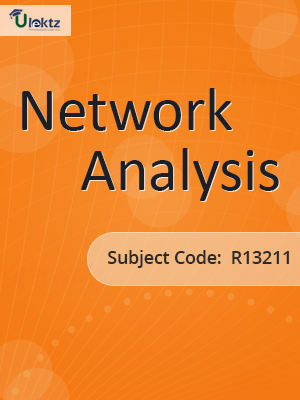•My WalletMy Order
•My Profile
•My Connections
•My Books
•My Videos
•My Tests
•My Calender
•My Messages
•My Shopping Cart
•My Orders
•Account Settings
•Help

# Book Details# Network Analysis

 Course Code : R13211 Author : uLektz University : JNTU Kakinada Regulation : 2016 Categories : Electronics & Communication Format :ePUB3 (DRM Protected) Type : eBook

FREE

Description :Network Analysis of R13211 covers the latest syllabus prescribed by JNTU Kakinada for regulation 2016. Author: uLektz, Published by uLektz Learning Solutions Private Limited.

Note : No printed book. Only ebook. Access eBook using uLektz apps for Android, iOS and Windows Desktop PC.

##### Topics
###### UNIT – I Introduction to Electrical Circuits-A.C Fundamentals and Network Topology

1.1 Introduction to Electrical Circuits : Network elements classification, Electric charge and current, Electric energy and potential, Resistance parameter – series and parallel combination, Inductance parameter – series and parallel combination, Capacitance parameter – series and parallel combination. Energy sources: Ideal, Non-ideal, Independent and dependent sources, Source transformation, Kirchoff’s laws, Mesh analysis and Nodal analysis problem solving with resistances only including depen

1.2 A.C Fundamentals and Network Topology: Definitions of terms associated with periodic functions: Time period, Angular velocity and frequency, RMS value, Average value, Form factor and peak factor- problem solving, Phase angle, Phasor representation, Addition and subtraction of phasors, mathematical representation of sinusoidal quantities, explanation with relevant theory, problem solving. Principal of Duality with examples.

1.3 Network Topology:Definitions of branch, node, tree, planar, non-planar graph, incidence matrix, Basic tie set schedule, basic cut set schedule

###### UNIT – II Steady State Analysis of A.C Circuits

2.1 Steady State Analysis of A.C Circuits: Response to sinusoidal excitation - pure resistance, pure inductance, pure capacitance

2.2 Impedance concept, phase angle, series R-L, R-C, R-L-C circuits problem solving

2.3 Complex impedance and phasor notation for R-L, R-C, R-L-C problem solving using mesh and nodal analysis

2.4 Star-Delta conversion, problem solving.

###### UNIT – III Coupled Circuits -Resonance

3.1 Coupled Circuits: Self inductance, Mutual inductance, Coefficient of coupling

3.2 Analysis of coupled circuits, Natural current, Dot rule of coupled circuits, Conductively coupled equivalent circuits- problem solving

3.3 Resonance: Introduction, Definition of Q, Series resonance, Bandwidth of series resonance

3.4 Parallel resonance, Condition for maximum impedance, current in anti resonance, Bandwidth of parallel resonance

3.5 General case- resistance present in both branches, anti resonance at all frequencies

###### UNIT – IV Network Theorems

4.1 Network Theorems: Thevenin’s, Norton’s

4.2 Milliman’s, Reciprocity, Compensation, Substitution

4.3 Superposition, Max Power Transfer, Tellegens- problem solving using dependent sources also.

###### UNIT – V Two-port networks

5.1 Two-port networks: Relationship of two port networks, Z-parameters, Y-parameters, Transmission line parameters, h-parameters, Inverse h-parameters, Inverse Transmission line parameters, Relationship between parameter sets

5.2 Parallel connection of two port networks, Cascading of two port networks, series connection of two port networks, problem solving including dependent sources also.

###### UNIT – VI Transients

6.1 Transients : First order differential equations, Definition of time constants, R-L circuit, R-C circuit with DC excitation, Evaluating initial conditions procedure, second order differential equations, homogeneous, non-homogeneous, problem solving using R-L-C elements with DC excitation and AC excitation, Response as related to s-plane rotation of roots. Solutions using Laplace transform method.